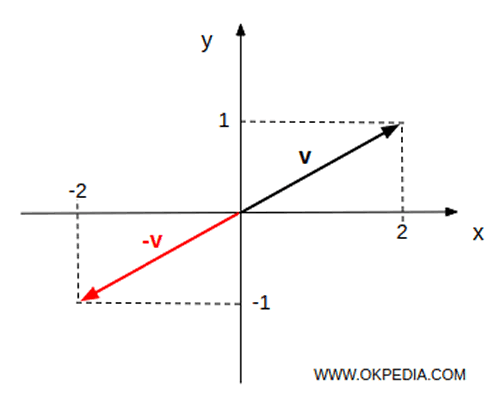# Negative vector

A negative vector -v is a vector that has the same magnitude as a vector v but in the opposite direction.

Example

Given a vector v

$$v = \begin{pmatrix} 2 \\ 1 \end{pmatrix}$$

The negative -v of the vector v is a vector obtained by the scalar product between -1 and the vector v

$$-v = -1 \cdot v = -1 \cdot \begin{pmatrix} 2 \\ 1 \end{pmatrix} = \begin{pmatrix} -2 \\ -1 \end{pmatrix}$$

The negative vector -v in R2 has the same length but in the opposite direction.www.okpedia.com - Okpedia - Contact email: info@okpedia.it - P.IVA - 09286581005 - Privacy Google - Disclaimer

Vectors

y